# PPP for hydrographyDecember 15, 2015  -A new high-accuracy technique using one dual-frequency GNSS receiver, precise point positioning (PPP) offers the possibility of cost-effectively obtaining coordinates. This study investigates the accuracy of kinematic PPP for hydrographic applications on rivers, and shows results comparable to double-difference solutions.

By Ashraf Abdallah and Volker Schwieger

Precise Point Positioning (PPP) is a challenging surveying technique for high-accuracy  results. It offers the advantage of using one dual-frequency GNSS instrument. Estimation of a PPP solution is based on the ionosphere-free linear combination for code data and carrier-phase data.

Bernese Software. Bernese software V. 5.2 is a GNSS post-processing software, using GNSS measurement data for static and kinematic surveying. It processes the data in double-difference (differential GNSS) and zero-difference (PPP solution) techniques. The software was developed at the Astronomical Institute of the University of Bern.

Bernese software contains a group of different tools or programs to complete the processing for double-difference or zero-difference mode. The estimation of the two techniques has the same processing schedule in most of the pre-processing stages. The change appears later within the parameter estimations section.

As shown in Figure 1, the processing starts with downloading the related orbits from the CODE (Center for Orbit Determination in Europe) FTP server. The orbit tools include the updating of the Earth orientation parameters to be in Bernese format, converting the satellite data to a specific format and generating the standard orbit format for Bernese software. A preprocessing program contains the smoothing of the RINEX data from outliers and cycle slips.

This smoothing step is following by converting the RINEX into Bernese binary format. The receiver clock is synchronized with respect to the GPS time and stored to observation files using clock synchronization tools. Using the code solution, a kinematic file is written to be inserted in the next parameter estimation procedure. For double-difference solution, a baseline is created, and this baseline is corrected from cycle slips for phase data. Parameter estimation is carried out by least-square estimation for the phase and code GNSS observations.

Kinematic PPP Solution. Bernese software provides the possibility to obtain the PPP solutions in automatic script (Bernese Protocol Engine [BPE]). The satellite orbit and clock ephemeris data from CODE center were used with intervals of 5 seconds to obtain highly accurate results. Satellite and receiver phase center offsets are considered. Tropospheric correction is applied using the Global Mapping Function (GMF) model for the hydrostatic and wet delay estimation. Regarding ionospheric correction, the estimation of the PPP solution is based on the linear ionospheric-free combination, with high-order ionospheric parameters to improve the estimation.

Figure 2 gives the analysis flowchart. Some outputs of the PPP solution could be visualized, such as the satellite phase and code residuals. The high residuals might come from the lower elevation angles of the satellites. Moreover, the residuals appear because of the effect of the remaining observation errors, such as atmospheric delay, multipath, or even the satellite orbit and clock residuals.

Regarding kinematic PPP solution, the error values in the east, north and ellipsoidal height are calculated with respect to the double-difference solution from Bernese software. The root-mean-square (RMS) error, which refers to the double-difference solution, and the standard deviation (SD), which is related to the mean value of the PPP solution error, are calculated, and the frequency histogram is plotted.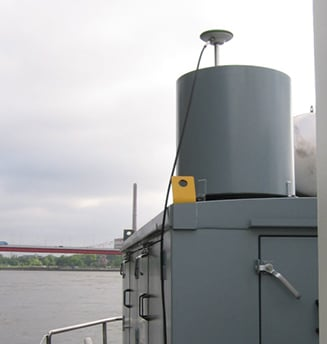An antenna and a receiver were mounted on the surveying vessel to collect the GNSS data with an interval of 1 second during two days.

Experimental Work. Two kinematic trajectories were observed on the Rhine River in Duisburg, Germany, as a part of the project “HydrOs — Integrated Hydrographical Positioning System.” The project was launched in cooperation with Department M5 (Geodesy) of the German Federal Institute of Hydrology (BfG) and the Institute of Engineering Geodesy at the University of Stuttgart (IIGS) .

An antenna and a receiver were mounted on the surveying vessel (inset photo, opener) to collect the GNSS data with an interval of 1 second during two days. The virtual SAPOS (SAtellitenPOSitionierungsdienst der deutschen Landesvermessung) reference station was considered as a reference station, provided from the SAPOS-NRW team. SAPOS is a continuously operating reference station (CORS) GNSS service collecting data throughout Germany.

### Results and Discussions

The layout of the first trajectory, which was observed for more than three hours, is presented in Figure 3. The measurements started from the inner harbour in Duisburg. The left figure shows the overview layout, and the right figure illustrates a zoom-in of the trajectory below two bridges. The white line refers to the kinematic PPP trajectory; the cross-hatched white line shows interpolated points between two solved points from the PPP solution. Because of loss of GNSS signals from the bridges, the yellow line indicates the actual vessel trajectory below bridges.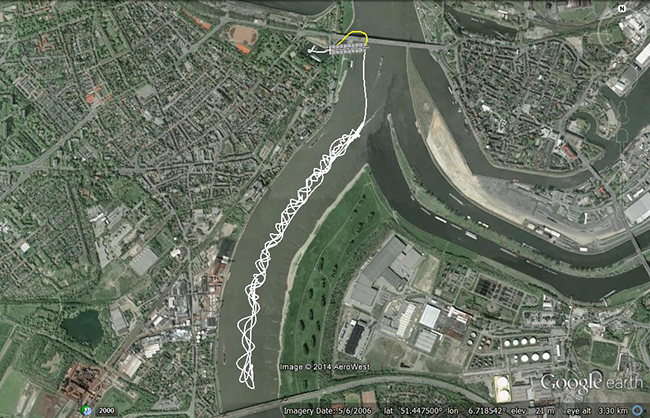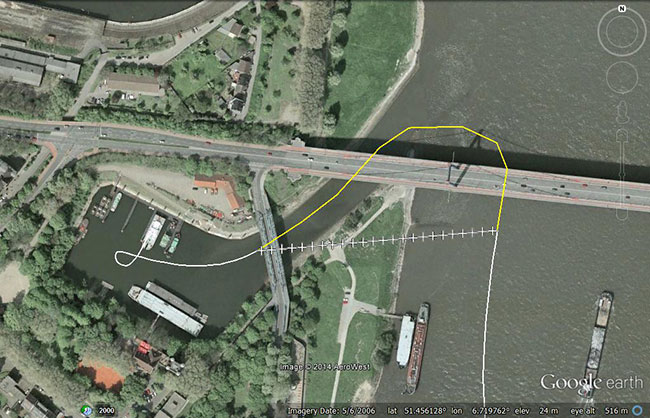Figure 3. Layout of the first trajectory [DOY: 2014/126], zoom-in on bottom. (Photos: Google Earth)

As mentioned before, the double-difference solution of the Bernese software is considered as the reference solution for the PPP solution. The PPP residuals for phase and code observations (not using double-difference solution) are presented in Figure 4. Here the residual values in phase and code have a gap because of the loss of GNSS signals, which starts from epoch 438 to 486 [GPS week second = 199845: 200115]. Additionally, there are some cycle slips from epoch 883 to 892 [GPS week second = 202105: 202150].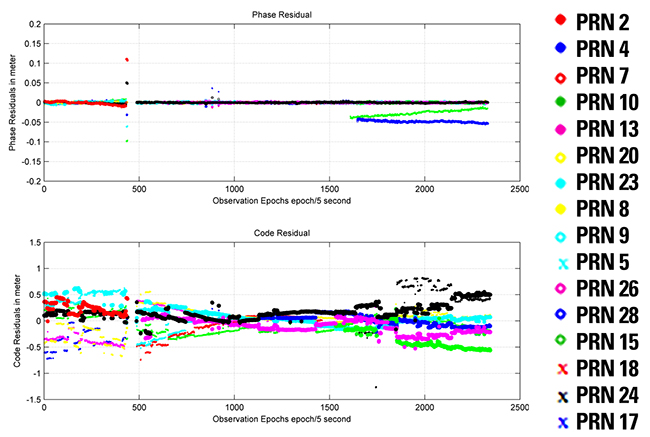Figure 4. Satellite residuals for the first trajectory [DOY: 2014/126].

To assess the accuracy of the PPP solution for this hydrographic trajectory, Figure 5 illustrates the analysis results for this trajectory between the double-difference and PPP solutions. The X-axis refers to the number of observations (one epoch/5 seconds), and the Y-axis indicates the error value in meters. Figure 5.1 shows the error plot (m) in east, north and height. As shown previously, the error values have a gap in the solution because of the loss of lock below the bridges. Moreover, there are some cycle slips later on, which decrease the estimated kinematic PPP accuracy.

Figures 5.2 and 5.3 provide the error plot for the east and north and east and height directions. The blue points refer to the errors, and the red cross refers to the mean value. Table 1 summarizes the PPP results.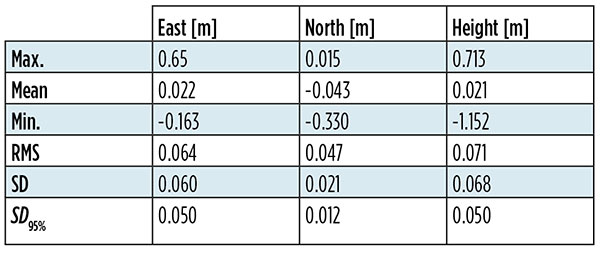Table 1. Statistical results of the first trajectory [DOY: 126/2014].

Five percent of the PPP errors are eliminated to get outlier-free results. The SD (95%) of the kinematic PPP solution is obviously improved to reach 5.0 cm, 1.20 and 5.0 cm in east, north and height directions, respectively.

To distinguish between the standard deviation and the standard deviation based on 95 percent of the data, Figure 5 shows additionally the histogram of SD in Figures 5.4, 5.5 and 5.6 for east, north and height respectively. Figures 5.7, 5.8 and 5.9 provide the error with 95 percent of the results. Absolutely, the error range is improved by eliminating 5 percent of the data including outliers.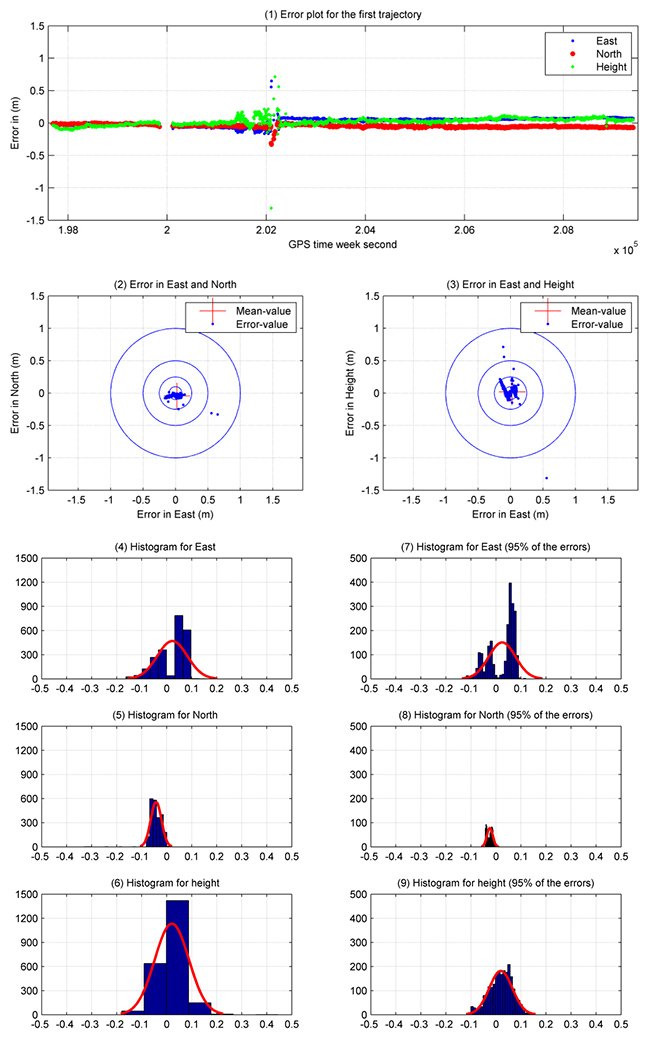Figure 5. Analysis results for the first trajectory. Standard deviations shown in plots on the left, with outliers excluded, right.

Second Data Set. The second trajectory on the Rhine River was observed [DOY: 127] for more than 5 hours (see Figure 6). Sixteen satellites were observed during the measurement time.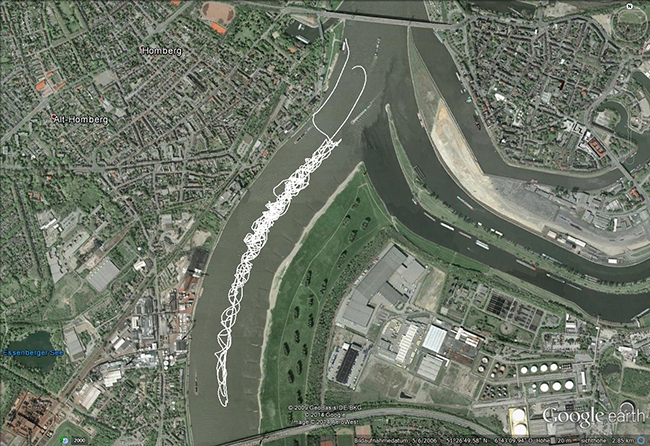Figure 6. Layout of the second trajectory [DOY: 127/2014]. (Photo: Google Earth)

In Figure 7, the phase and code residuals are plotted. Some outliers are reported in this graph, which refers to cycle slips during the observations.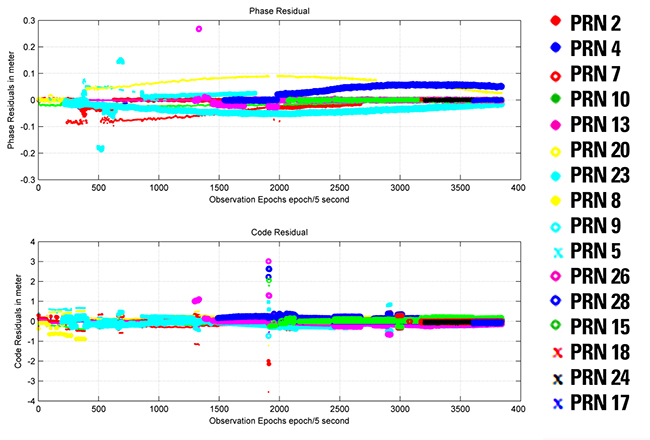Figure 7. Satellite residuals for the second trajectory [DOY: 127/2014].

Figure 8 illustrates the PPP results for this kinematic trajectory. Figure 8.1 shows the PPP error values in the east, north and height directions with respect to the double-difference solution from Bernese software.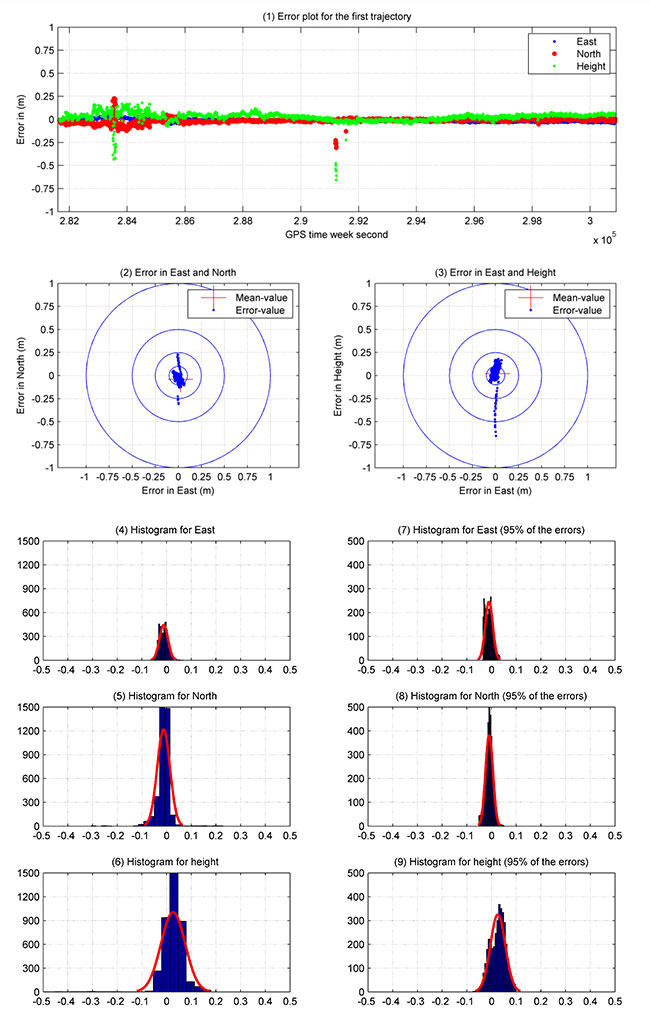Figure 8. Kinematic PPP solution for the second trajectory. Standard deviations shown in plots on the left, with outliers excluded, right.

The first 40 minutes of that trajectory were realized in a quasi-static observation technique (nonmoving vessel) from GPS week second 281660: 284060. The result obtained from this solution is more accurate due to the high number of satellites, and the trajectory did not include the bridges area. Figure 8.2 and 8.3 show errors in east and north, and east and height.

As shown in Table 2, the maximum and minimum values for the error range, which are presented in detail in Figure 8.4, 8.5 and 8.6, are reported in the east, north and height directions. These figures show the frequency histogram for the PPP errors. The RMS error from the solution is 2.10 cm and 2.90 cm in east and north respectively, with an RMS error of 5.60 cm in height. The standard deviation is definitely improved after eliminating 5 percent of the PPP errors as outliers. The standard deviation for 95 percent of the results shows 1.5 cm in east and north and 3 cm in height. The error histograms for 95 percent of the data are provided in Figures 8.7, 8.8 and 8.9.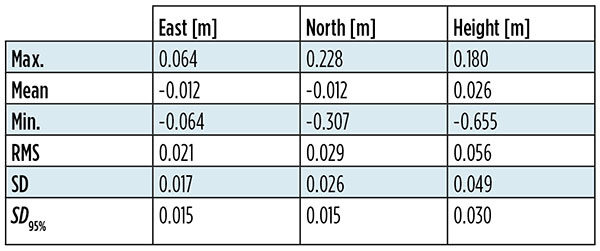Table 2. Statistical results of the second trajectory [DOY: 127/2014].

The second trajectory clearly provides a higher accuracy than the first. Its data has a higher number of satellites and lower outliers than the first. Figure 8 shows the histogram of the second trajectory is similar to the Gaussian distribution curve.

### Acknowledgments

The authors would like to thank Annette Scheider for receiving the GNSS measurements through the HydrOs project, our BfG partners Harry Wirth and Marc Breitenfeld, and Bernhard Galitzki form SAPOS-NRW for providing us with the reference stations.

### Manufacturers

A Leica 1203+ antenna and GX1230+ GNSS receiver collected the data shown here.

Ashraf Abdallah is an assistant lecturer in engineering, Aswan University, Egypt, and a Ph. D. student at the Institute of Engineering Geodesy (IIGS), Stuttgart University, Germany. He received a master’s degree from Aswan University in applications of single-frequency GNSS.

Volker Schwieger is a full professor at the University of Stuttgart and director of the IIGS. He received a Ph.D. from the University of Hannover, focusing on GPS for monitoring applications.